5.2.2 LP filter coding and interpolation

26.4453GPPCodec for Enhanced Voice Services (EVS)Detailed algorithmic descriptionRelease 15TS

5.2.2.1 LSF quantization

5.2.2.1.1 LSF weighting function

For frame-end LSF quantization, the weighting given by equation (473) is defined by combining the magnitude weighting, frequency weighting, IHM and squared IHM.

As shown in figure 1, since the spectral analysis and LP analysis use similar temporal sections, the FFT spectrum of the second analysis window can be reused to find the best weighting function for the frame-end LSF quantizer.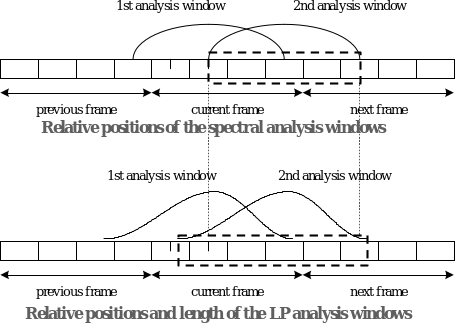Figure 19: LSF weighting computation with FFT spectrum

Figure 2 is a block diagram of a spectral analysis module that determines a weighting function. The spectral analysis computation is performed by a pre-processing module and the output is a linear scale spectrum magnitude which is obtained by FFT.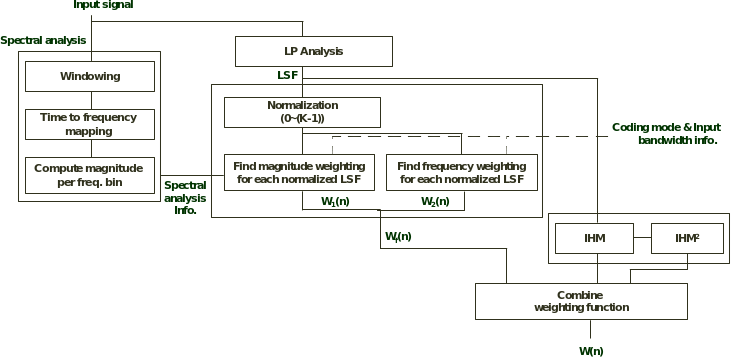Figure 20: Block diagram of LSF weighting computation

In the Normalization block, the LSFs are normalized to a range of 0 to -1. The LSFs generally span the range of 0 to . For a 12.8 kHz internal sampling frequency, is 128 and for a 16 kHz internal sampling frequency, is 160.

The Find magnitude weighting for each normalized LSF block determines the magnitude weighting function using the spectrum analysis information and the normalized LSF.

The magnitude weighting function is determined using the magnitude of the spectral bins corresponding to the frequency of the normalized LSFs and the additional two magnitudes of the neighbouring spectral bins (+1 and -1 of the spectral bin corresponding to the frequency of the normalized LSFs) around the spectral bin.

The spectral magnitude is obtained by a 128-point FFT and its bandwidth corresponds to the range of 0 to 6400 Hz. If the internal sampling frequency is16 kHz, the number of spectral magnitudes is extended to 160. Because the spectrum magnitude for the range of 6400 to 8000 Hz is missing, the spectrum magnitude for this range will be generated by the input spectrum. More specifically, the average value of the last 32 spectrum magnitudes which correspond to the bandwidth of 4800 to 6400 Hz are repeated to fill in the missing spectrum.

The final magnitude function determines the weighting function of each magnitude associated with a spectral envelope by extracting the maximum magnitude among the three spectral bins.

,for n=0,…,M-1 (473)

where Min is the minimum value of and

, for n=0,…,M-1 (474)

where =16 and the is the maximum magnitude among the three spectral bins for each LSF.

In the Find frequency weighting for each normalized LSF block, the frequency weighting function is determined by using frequency information from the normalized LSF.

The function determines the weighting function of each frequency using the predetermined weighting graph which is selected by using the input bandwidth and coding mode. There are two predetermined weighting graphs, as shown in figure 3, which are determined by perceptual characteristics such as Bark scale and a formant distribution of the input signal.

The function corresponding to graph (a) in figure 4 is as follows.

(475)

The function corresponding to graph (b) in figure 21 is as follows.

(474)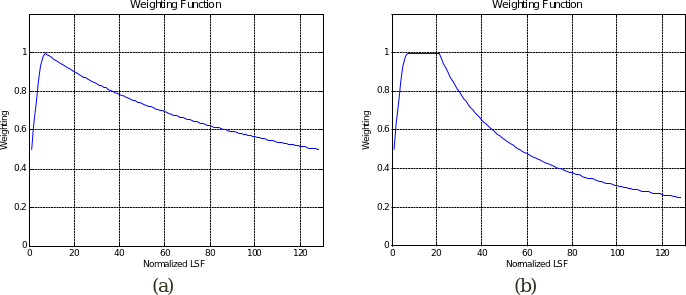Figure 21: Frequency weighting functions

Next, the FFT weighting function is determined by combining the magnitude weighting function and the frequency weighting function. Computing the FFT weighting function for frame-end LSF quantization is performed as follows:

, n=0,…,M-1 (477)

The FFT weighting function uses different types of frequency and magnitude weighting functions depending on frequency bandwidth (NB, WB or WB16 kHz) and coding modes (UC or others such as VC, GC, AC, IC and TC).

Along with the FFT weightings , another weighting function called the inverse harmonic mean (IHM) is computed and defined as:

, n=0,…,M-1 (478)

The LSFs are normalized between 0 and , where the first and the last weighting coefficients are calculated with this pseudo LSFs and . M is the order 16 of the LP model.

IHM approximates the spectral sensitivity of LSFs by measuring how close adjacent LSFs come. If two LSF parameters are close together the signal spectrum has a peak near that frequency. Hence a LSF that is close to one of its neighbours has a high scalar sensitivity and should be given a high weight. The sensitivity of close neighbours LSF is even enhanced by computing the squared of IHM:

, n=0,…,M-1 (479)

The three set of weightings, , , and are gathered into an M by 4 matrix as follows:

(480)

The set of weightings are combined linearly by multiplying the matrix E by a constant column vector P of dimension M:

(481)

The vector P is different for NB, WB/SWB at internal sampling rate 12.8 kHz and WB/SWB at internal sampling rate 16 kHz. The vectors P are derived off-line over a training data by minimizing the distance of the linear combination W and the weightings derived mathematically based on Gardner and Rao method, weightings near-optimal but too complex for being computed on-line compared to an heuristic approach.

5.2.2.1.2 Bit allocation

The frame-end LSF quantization codebooks and bit allocations depend on the selected coding mode. In addition, different codebooks are used for NB, WB and WB 16kHz modes. This means there is a separate, optimized codebook for each coding mode and for each input bandwidth. In NB mode the LSF vectors are in the range of 0-6400Hz although the input signal has content only up to 4kHz. The WB mode corresponds to the mode where the LSF parameters are estimated in the 0-6400Hz range. The WB2 mode corresponds to the mode where the LSF parameters are estimated in the 0-8000Hz range and it is used in general for the higher bitrates.

Table 1 shows the bit allocation for frame-end LSF quantization for each coding mode.

Table 23: Bit allocation for LSF vectors

 ACELP corebitrate (kbps) Inactive Unvoiced Voiced Generic Transition Audio 3.6 0 27 16 22 0 0 7.2 22 37 31 29 31 22 8.0 22 40 36 33 34 22 9.6 31 31 31 31 0 0 13.20 31 0 38 38 40 31 16.40 31 0 31 31 0 31 24.40 31 0 31 31 0 31 32.00 41 0 0 41 41 0 64.00 41 0 0 41 41 0
5.2.2.1.3 Predictor allocation

There are three possible cases. In safety net only the mean removed LSF vectors are quantized with the multi stage quantizer. In MA predictive quantization the MA prediction error is quantized with the MSVQ. In switched safety net /AR predictive there is a selection between quantizing the mean removed LSF vector and the AR prediction error. Table 24 specifies for each coding type and each bandwidth which quantization scheme is used. The values in the table indicate safety net (0), MA prediction (1), and AR prediction combined with safety net (2). The value “-1“ indicates that the corresponding mode is not used. The coding modes that employ switched safety net/ AR prediction use one bit to signal which one of the two variants is used.

Table 25: Predictive mode type for LSF quantizer

 Inactive Unvoiced Voiced Generic Transition Audio Narrowband 1 1 2 2 0 2 Wideband <9.6kbps 1 1 2 2 0 2 Wideband 16kHz 1 -1 2 1 0 1 Wideband >=9.6kbps 1 1 2 1 0 1

The predictor values are optimized for all quantizer modes. For a given coding mode and bandwidth, all bitrates use the same predictor values. In general LSF values for voiced speech are considered quite stable over several consecutive frames. Consequently the corresponding AR predictor has the highest coefficient values. Other AR predictor coefficients are slightly lower. For the MA predictor the same value of 1/3 is used everywhere. The value is significantly lower than for AR coefficients since the quantization error starts oscillating over time if the MA coefficient is too large. The value is experimentally chosen to provide reasonable prediction efficiency, stability and good error recovery.

5.2.2.1.4 LSF quantizer structure

A safety net, predictive or switched safety-net predictive multi-stage vector quantizer (MSVQ) is used to quantize the full length frame-end LSF vector for all modes except voiced mode at 16 kHz internal sampling frequency. The last stage of the MSVQ is a multiple scale lattice vector quantizer (MSLVQ) . For each coding mode number of 1 to 4 unstructured VQ stages are used followed by a MSLVQ stage. The number of stages, number of bits per each stage and the codebook names for each coding mode are detailed in table 26. The codebook names are mentioned to illustrate how some of the codebooks are reused between modes.

Table 27: Optimized codebooks and their bit allocation for LSF quantizers

 Coding mode Bits VQ safety net Bits in VQ stages – safety net Codebooks Bits VQ predictive mode Bits in VQ stages predictive mode Codebooks Inactive NB – – – 5 5 IAA_MA1 Unvoiced NB – – – 8 4+4 UVD_MA1UVD_MA2 Voiced NB 8 4+4 SVNB_SN1SVNB_SN2 6 3+3 GESVNB_AR1GESVNB_AR2 Generic NB 9 5+4 GETRNB_SN1GETRNB_SN2 6 3+3 GESVNB_AR1GESVNB_AR2 Transition NB 9 5+4 GETRNB_SN1GETRNB_SN2 – – – Audio NB 4 4 AUNB_SN1 0 0 – Inactive WB – – – 5 5 IAA_MA1 Unvoiced WB – – – 12 4+4+4 UVD_MA1UVD_MA2UVWB_MA3 Voiced WB 8 4+4 SVWB_SN1SVWB_SN2 6 3+3 GESVWB_AR1GESVWB_AR2 Generic WB 9 5+4 GETRWB_SN1GETRWB_SN2 6 3+3 GESVWB_AR1GESVWB_AR2 Transition WB 9 5+4 GETRWB_SN1GETRWB_SN2 – – – Audio WB 4 4 AUWB_SN1 0 0 – Inactive WB2 – – – 5 5 IAA_MA1 Unvoiced WB2 – – – – – – Voiced WB2 – – BC-TCVQ – – BC-TCVQ Generic WB2 – – – 5 5 GEWB2_MA1 Transition WB2 8 4+4 TRWB2_SN1TRWB2_SN2 – – – Audio WB2 – – – 5 5 AUWB2_MA1 CNG 4 4 CNG_SN1 – – – Generic WB >= 9.6kbps – – – 5 5 GEWB_MA1

The WB2 voiced mode is using BC-TCVQ technology detailed in subclause 5.2.2.1.5.

Overall the optimized VQ codebooks use 14,368 kBytes and the MSLVQ parameters use 9.304 kBytes, including CNG mode.

The remaining LSF quantizer bits are used for the MSLVQ stage. The quantization in all the stages is done such that it minimizes a weighted Euclidean distortion. The calculation of the weights is detailed in subclause 5.2.2.2.1. The search in the multi-stage quantizer is done such that at most 2 candidates are kept per stage. For each candidate obtained in the search in the unstructured optimized VQ, a residual LSF vector is formed by subtracting from the LSF vector the codevectors obtained in each unstructured VQ stage. If there is one optimized VQ stage two residual LSF vectors are obtained, if there are two optimized VQ stages, 4 candidates are obtained and so on.

Each residual LSF vector is split into two 8-dimensional sub vectors. Each sub vector is coded as follows. The lattice codebook obtained through the reunion of three D8+ lattice truncations differently scaled. Each lattice truncation has a different number of leader classes. The leader classes contained in the lattice truncations are given in table 28.

Table 29: Lattice leader class vectors

 Leader class index Leader class vector Leader class index Leader class vector 0 1.0, 1.0, 0.0, 0.0, 0.0, 0.0, 0.0, 0.0 25 3.0, 1.0, 1.0, 1.0, 1.0, 1.0, 0.0, 0.0 1 0.5, 0.5, 0.5, 0.5, 0.5, 0.5, 0.5, 0.5 26 3.0, 2.0, 1.0, 0.0, 0.0, 0.0, 0.0, 0.0 2 1.0, 1.0, 1.0, 1.0, 0.0, 0.0, 0.0, 0.0, 27 1.5, 1.5, 1.5, 1.5, 1.5, 1.5, 0.5, 0.5 3 2.0, 0.0, 0.0, 0.0, 0.0, 0.0, 0.0, 0.0 28 2.5, 1.5, 1.5, 1.5, 0.5, 0.5, 0.5, 0.5 4 1.5, 0.5, 0.5, 0.5, 0.5, 0.5, 0.5, 0.5 29 2.5, 2.5, 0.5, 0.5, 0.5, 0.5, 0.5, 0.5 5 1.0, 1.0, 1.0, 1.0, 1.0, 1.0, 0.0, 0.0 30 3.5, 0.5, 0.5, 0.5, 0.5, 0.5, 0.5, 0.5 6 2.0, 1.0, 1.0, 0.0, 0.0, 0.0, 0.0, 0.0 31 2.0, 2.0, 2.0, 1.0, 1.0, 1.0, 1.0, 0.0 7 1.5, 1.5, 0.5, 0.5, 0.5, 0.5, 0.5, 0.5 32 2.0, 2.0, 2.0, 2.0, 0.0, 0.0, 0.0, 0.0 8 1.0, 1.0, 1.0, 1.0, 1.0, 1.0, 1.0, 1.0 33 3.0, 1.0, 1.0, 1.0, 1.0, 1.0, 1.0, 1.0 9 2.0, 1.0, 1.0, 1.0, 1.0, 0.0, 0.0, 0.0 34 3.0, 2.0, 1.0, 1.0, 1.0, 0.0, 0.0, 0.0 10 2.0, 2.0, 0.0, 0.0, 0.0, 0.0, 0.0, 0.0 35 4.0, 0.0, 0.0, 0.0, 0.0, 0.0, 0.0, 0.0 11 1.5, 1.5, 1.5, 0.5, 0.5, 0.5, 0.5, 0.5 36 1.5, 1.5, 1.5, 1.5, 1.5, 1.5, 1.5, 0.5 12 2.5, 0.5, 0.5, 0.5, 0.5, 0.5, 0.5, 0.5 37 2.5, 1.5, 1.5, 1.5, 1.5, 0.5, 0.5, 0.5 13 2.0, 1.0, 1.0, 1.0, 1.0, 1.0, 1.0, 0.0 38 2.5, 2.5, 1.5, 0.5, 0.5, 0.5, 0.5, 0.5 14 2.0, 2.0, 1.0, 1.0, 0.0, 0.0, 0.0, 0.0 39 3.5, 1.5, 0.5, 0.5, 0.5, 0.5, 0.5, 0.5 15 3.0, 1.0, 0.0, 0.0, 0.0, 0.0, 0.0, 0.0 40 2.0, 2.0, 2.0, 2.0, 1.0, 1.0, 0.0, 0.0 16 1.5, 1.5, 1.5, 1.5, 0.5, 0.5, 0.5, 0.5 41 3.0, 2.0, 1.0, 1.0, 1.0, 1.0, 1.0, 0.0 17 2.5, 1.5, 0.5, 0.5, 0.5, 0.5, 0.5, 0.5 42 3.0, 2.0, 2.0, 1.0, 0.0, 0.0, 0.0, 0.0 18 2.0, 2.0, 1.0, 1.0, 1.0, 1.0, 0.0, 0.0 43 3.0, 3.0, 0.0, 0.0, 0.0, 0.0, 0.0, 0.0 19 2.0, 2.0, 2.0, 0.0, 0.0, 0.0, 0.0, 0.0 44 4.0, 1.0, 1.0, 0.0, 0.0, 0.0, 0.0, 0.0 20 3.0, 1.0, 1.0, 1.0, 0.0, 0.0, 0.0, 0.0 45 1.5, 1.5, 1.5, 1.5, 1.5, 1.5, 1.5, 1.5 21 1.5, 1.5, 1.5, 1.5, 1.5, 0.5, 0.5, 0.5 46 2.5, 1.5, 1.5, 1.5, 1.5, 1.5, 0.5, 0.5 22 2.5, 1.5, 1.5, 0.5, 0.5, 0.5, 0.5, 0.5 47 2.5, 2.5, 1.5, 1.5, 0.5, 0.5, 0.5, 0.5 23 2.0, 2.0, 1.0, 1.0, 1.0, 1.0, 1.0, 1.0 48 3.5, 1.5, 1.5, 0.5, 0.5, 0.5, 0.5, 0.5 24 2.0, 2.0, 2.0, 1.0, 1.0, 0.0, 0.0, 0.0 49

Given the bitrate available for the lattice codebook, the codebook is thus defined by a set of three integers representing the number of leader vectors for each truncation and three positive real number representing the scale for each lattice truncation. For instance a multiple scale lattice structure is defined by the number of leaders (20, 14, 5, 16, 10, 0) and the scales (1.057, 1.794, 2.896, 1.154, 1.860, 0.0). It means that the first subvector is quantized with a structure having three lattice truncations having 20, 14, and 5 leader classes respectively, which are scaled with the scales 1.057, 1.794, 2.896, respectively. The second subvector has only two truncations having 16 and 10 leader classes respectively. The truncations are ordered such that, for each subvector, their number of leader classes is descendingly ordered.

The difference in number of bits between the total number of bits for LSF end encoding, the prediction bit if needed and the number of bits used for unstructured VQ is used for the MSLVQ stage. The quantization in all the stages is done such that it minimizes a weighted Euclidean distortion. The calculation of the weights is detailed in subclause 5.2.2.1.1.

Suppose is the current LSF 8-dimensional sub vector and w its corresponding weight vector. The vector is normalized, i.e. component wise multiplied with the inverse of the off line estimated standard deviation. The resulting vector is further sorted in descending order based on the absolute value of its components and the weights vector is arranged following the same order. Let be the vector of descendingly sorted absolute values of and the correspondingly sorted weights vector. The weighted distance to the best codevector of each leader class corresponds to:

(475)

where is the leader vector corresponding to class and is the scale of the truncation . Each lattice codebook has at most 3 truncations with their corresponding scales. Each truncation has a given number of leader vector classes. The sum of cardinalities of the classes for the truncations forming the codebook for the first LSF subvector and for the second subvector are within the number of bits for the considered operating point given by the overall bitrate and bandwidth. Computing in the transformed input space the second and the third terms from equation (476) directly gives a relative measure of goodness for the best codevector from the leader class and truncation which may be considered as a potential codevector for the truncation and the leader class .

(477)

The part of equation (478) that is independent of the scale is calculated only once for all the leader classes from the first truncation, which is the one having the highest number of leader classes. When adding the last term to the first sum of equation (483) the product is considered with negative sign if the parity constraint of the leader is not obeyed by the signs of the vector . The contribution of the scale values is considered only afterwards in order to obtain the value . The leader class vector and the truncation j having the smallest correspond to the codevector of the current input vector. The inverse permutation of the sorting operation on the input vector applied on the winning leader vector gives the lattice codevector after applying also the corresponding signs. If the parity of leader vector is 0 the signs are identical to the signs of the input vector. If the parity is 1 the signs are similar to the signs of the input vector with the constraint that the number of negative components is even. If the parity is -1 the signs are similar with signs of the input vector with the constraint that the number of negative components is odd. The final codevector is obtained after multiplication with the scale and with the inverse of the component-wise off-line computed standard deviation. The standard deviations are individually estimated for each coding mode and bandwidth.

The candidate quantized LSF vectors are obtained by adding each lattice quantized residual to the corresponding candidates from the upper stages. The obtained candidates are increasingly sorted. For each sorted candidate the weighted Euclidean distortion with respect to the original LSF vector is calculated. The candidate that minimizes this distortion is selected as codevector to be encoded. The indexes corresponding to the first unstructured optimized VQ codebooks together with the index in the lattice codebook are written in the bitstream. The index for the lattice codebook is obtained as described in subclause 5.2.2.1.4.2.

For the CNG mode, using a total of 29 bits for the LSF quantization, the multiple scale lattice codebook structure is specific to each of the 16 codevectors obtained in the first stage. In addition based on the value of the last component of the 16 dimensional LSF vector only part of the first stage codebook is searched. If the last component of is larger than 6350 then the search is done only for the first 6 codevectors of the first stage and the LSF vector corresponds to internal sampling frequency of 16kHz, otherwise the search is performed within the last 10 codevectors of the first stage.

5.2.2.1.4.1 Selection between safety net and predictive mode

For the modes where switched safety-net prediction is allowed the selection between the two is done as follows. For frame error concealment reasons safety net is imposed, and variable set to 1, under the following conditions:

– first three ACELP frames after an HQ frame

– in voiced class signals, if the frame erasure mode LSF estimate of the next frame based on the current frame is at a distance from the current frame LSF vector larger than 0.25. The distance, or stability factor, is calculated as:

(479)

where frame_len is the frame length of the current frame and D is the Euclidean distance between the current frame LSF vector and the FER estimate for the next frame. In this case calculated at the current frame is stored in memory for use at the subsequent frame, thereby forcing the safety net decision for the subsequent frame when is equal to 1.

– some cases of rate switching

Safety net usage is decided by the following code line:

if ( force_sf || Err < abs_threshold || Err*(*streaklimit) < 1.05 * Err)

Thus the safety net mode is selected if force_sf is enabled or if for the quantized safety net codevector the quantization distortion (weighted Euclidean distance) is smaller than abs_threshold of 41000 for NB or 45000 for WB frames. For these relatively low error values the quantization is already transparent to original LSF values and it makes sense from the error recovery point of view to use safety-net as often as possible. Finally the safety net quantized error is compared to the predictively quantized error, with scaling of 1.05 to prefer safety net usage as well using *streaklimit multiplying factor that is adaptive to the number of consecutive predictive frames. The *streaklimit factor gets smaller, when the streak of continuous predictive frames gets longer. This is done in order to restrict the very long usage streaks of predictive frames for frame-erasure concealment reasons. For voiced speech longer predictive streaks are allowed than for other speech types. In voiced mode streak limiting starts after 6 frames, in other modes after 3 frames.

5.2.2.1.4.2 Indexing of the lattice codevector

The indexes of each one of the two multiple scale lattice codevectors is composed of the following entities:

– scale indexes for the two 8-dimensional subvectors

– leader class index, for the two 8-dimensional subvectors

– leader permutation index, unsigned permutation index

– scale offset the number of codevectors corresponding to the truncations with smaller scale indexes

– , i=1,2 cardinality of unsigned leader class, i.e. number of unsigned permutations in the class, shown in table 30.

– is the number of codevectors for the second subvector

The index for each subvector is calculated using

(480)

The indexes Ili and Isi are obtained using the position encoding based on counting the binomial coefficients and the sign encoding described in .

Table 31: Cardinality of unsigned leader vector permutations

 Leader vector index Leader vector index Leader vector index 0 28 17 56 34 1120 1 1 18 420 35 8 2 70 19 56 36 8 3 8 20 280 37 280 4 8 21 56 38 168 5 28 22 168 39 56 6 168 23 28 40 420 7 28 24 560 41 336 8 1 25 168 42 840 9 280 26 336 43 28 10 28 27 28 44 168 11 56 28 280 45 1 12 8 29 28 46 168 13 56 30 8 47 420 14 420 31 280 48 168 15 56 32 70 16 70 33 8

The binomial encoding used for calculating Il1 and Il2 uses the fact that the cardinality of an unsigned leader class with distinct values v0,…,vn-1, each having the number of occurrences k0,…,kn-1 is given by:

. (481)

The distinct values for each leader class vector and the number of each value in each leader class vector are given in the following table:

Table 32: Leader vector distinct values, their number of occurrences, and leader vector parities

 Leader class index Distinct values Number of occurrences Parity Leader class index Distinct values Number of occurrences Parity 0 1, 0, 2,6 0 25 3.0, 1.0, 0.0 1,5,2 0 1 0.5 8 1 26 3.0, 2.0, 1.0, 0.0 1,1,1,5 0 2 1, 0 4,4 0 27 1.5, 0.5 6,2 1 3 2, 0 1,7 0 28 2.5, 1.5, 0.5, 1,3,4 -1 4 1.5, 0.5 1,7 -1 29 2.5, 0.5 2,6 1 5 1.0, 0.0 6,2 0 30 3.5, 0.5, 1,7 -1 6 2.0, 1.0, 0.0, 1,2,5 0 31 2.0, 1.0, 0.0 3,4,1 0 7 1.5, 0.5 2,6 1 32 2.0, 0.0 4,4 0 8 1.0 8 0 33 3.0, 1.0 1,7 0 9 2.0, 1.0, 0.0 1,4,3 0 34 3.0, 2.0, 1.0, 0.0 1,1,3,3 0 10 2.0, 0.0 2,6 0 35 4.0, 0.0, 1,7 0 11 1.5, 0.5 3,5 -1 36 1.5, 0.5 7,1 -1 12 2.5, 0.5 1,7 1 37 2.5, 1.5, 0.5 1,4,3 1 13 2.0, 1.0, 0.0 1,6,1 0 38 2.5, 1.5, 0.5 2,1,5 -1 14 2.0, 1.0, 0.0 2,2,4 0 39 3.5, 1.5, 0.5 1,1,6 1 15 3.0, 1.0, 0.0 1,1,6 0 40 2.0, 1.0, 0.0 4,2,2 0 16 1.5, 0.5 4,4 1 41 3.0, 2.0, 1.0, 0.0 1,1,5,1 0 17 2.5, 1.5, 0.5 1,1,6 -1 42 3.0, 2.0, 1.0, 0.0 1,2,1,4 0 18 2.0, 1.0, 0 2,4,2 0 43 3.0, 0.0 2,6 0 19 2.0, 0.0 3,5 0 44 4.0, 1.0, 0.0 1,2,5 0 20 3.0, 1.0, 0.0, 1,3,4 0 45 1.5 8 1 21 1.5, 0.5 5,3 -1 46 2.5, 1.5, 0.5 1,5,2 -1 22 2.5, 1.5, 0.5 1,2,5 1 47 2.5, 1.5, 0.5 2,2,4 1 23 2.0, 1.0 2,6 0 48 3.5, 1.5, 0.5 1,2,5 -1 24 2.0, 1.0, 0.0 3,2,3 0 49

The index for the two multiple scale lattice codevectors corresponding to the two residual LSF subvectors are combined in a single index, I, which is written in the bitstream.

. (482)

5.2.2.1.5 LSFQ for voiced coding mode at 16 kHz internal sampling frequency : BC-TCVQ

5.2.2.1.5.1 Block-constrained trellis coded vector quantization (BC-TCVQ)

The VC mode operating at 16 kHz internal sampling frequency has two decoding rates: 31 bits per frame and 40 bits per frame. The VC mode is quantized by a 16-state and 8 stage block-constrained trellis coded vector quantization (BC-TCVQ) scheme.

Trellis coded vector quantization (TCVQ)  generalizes trellis coded quantization (TCQ) to allow vector codebooks and branch labels. The main feature of TCVQ is the partitioning of an expanded set of VQ symbols into subsets and the labelling of the trellis branches with these subsets. TCVQ is based on a rate-1/2 convolutional code, which has trellis states and two branches entering/leaving each trellis state. Given a block of m source vectors, the Viterbi algorithm (VA) is used to find the minimum distortion path. This encoding procedure allows the best trellis path to begin in any of N initial states and end in any of N terminal states. In TCVQ, the codebook has vector codewords. is referred to as “codebook expansion factor” (in bits per dimension) since the codebook has times as many codewords as a nominal rate- VQ. The encoding is accomplished in the following two steps.

Step 1. For each input vector, find the closest codeword and corresponding distortion in each subset.

Step 2. Let the branch metric for a branch labelled with subset S be the distortion found in step 1 and use the VA to find the minimum distortion path through the trellis.

BC-TCVQ is a low-complexity approach that requires exactly one bit per source sample to specify the trellis path. Figure 5 shows the concept of ‘block constrained’ and illustrates the search process of the Viterbi algorithm with a 4-state and 8 stages trellis structure, which selects ‘00’ and ‘10’ as initial states. When the initial state is ‘00’, the terminal state is selected to be one of ‘00’ or ‘01’ and when the initial state is ‘10’, the terminal state is selected to be one of ‘10’ or ‘11’. As an example, the survival path from the initial stage with state ‘00’ to the stages with state ‘00’ is shown by a dotted line. In this case, the only two possible trellis paths for the last two stages are toward states ‘00’ and ‘01’. This example uses one bit for the initial state and one bit for the terminal state. If the terminal state is decided, the path information for the last two stages is not needed.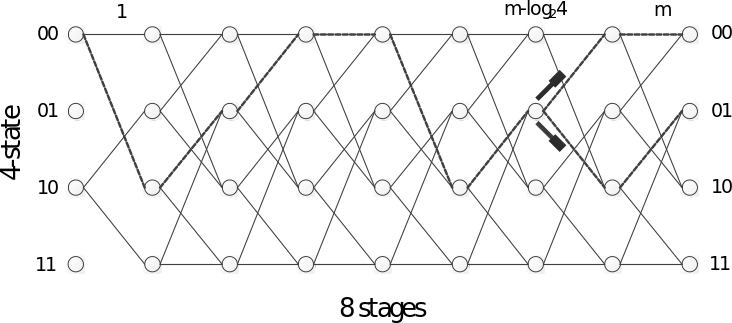Figure 22: Block constrained concept in 4-state and 8 stages trellis structure for BC-TCVQ encoding

For any , consider a BC-TCVQ structure that allows initial trellis states and exactly terminal trellis states for each allowed initial trellis state. A single VA encoding, starting from the allowed initial trellis states, proceeds in the normal way up to the vector stage . It takes k bits to specify the initial state, and bits to specify the path to vector stage . A unique terminating path, possibly dependent on the initial trellis state, is pre-specified for each trellis state at vector stage through vector stage . Regardless of the value of , the encoding complexity is only a single VA search of the trellis, and exactly m bits are required to specify an initial trellis state and a path through the trellis.

The BC-TCVQ for VC mode at a 16kHz internal sampling frequency utilizes 16-state (=16) and 8-stage (=8) TCVQ with 2-dimensional (=2) vector. LSF subvectors with two elements are allocated to each stage. Table 33 shows the initial states and terminal states for 16-state BC-TCVQ. In this case the parameters and are 2 and 4, respectively. Four bits are used for both the initial state and terminal state.

Table 34: Initial state and terminal state for 16-state BC-TCVQ

 Initial state Terminal state 0 0, 1, 2, 3 4 4, 5, 6, 7 8 8, 9, 10, 11 12 12, 13, 14, 15

5.2.2.1.5.2 Bit Allocations and codebook size for BC-TCVQ

The bit allocations for the LSF quantizer at 31 and 40 bits/frame are summarized in tables 35 and 36.

Table 37: Bit allocation for the LSF quantizer at 31 bits/frame

 Parameters Bit allocation BC-TCVQ Path information(Initial states + path + final states) 2+4+2 Subset codewords 4 bits 2 (Stages 1 to 2)3 bits 2 (Stages 3 to 4)2 bits 4 (Stages 5 to 8) Scheme selection 1 Total 31

Table 38: Bit allocation for the LSF quantizer at 40 bits/frame

 Parameters Bit allocations BC-TCVQ Path information(Initial states + path + final states) 2+4+2 Subset codewords 4 bits 2 (Stages 1 to 2)3 bits 2 (Stages 3 to 4)2 bits 4 (Stages 5 to 8) SVQ Subset codewords 5 (1st vector with dim.=8)4 (2nd vector with dim.=8) Scheme selection 1 Total 40

Figures 6 and 7 show the LSF quantizer at 31 and 40 bits/frame, respectively. The 1st and 2nd BC-TCVQ use the same bit allocation but different codebook entries. The 3rd and 4th SVQ use the same bit allocation and codebooks. The 31 bit LSF quantizer uses BC-TCVQ and the 40 bit LSF quantizer uses both BC-TCVQ and SVQ.

The following table summarizes the codebook size for BC-TCVQ and SVQ. The overall codebook size is 2,432 words. In addition, there are several tables for BC-TCVQ such as intra-prediction coefficients (56 words), scale information (32 words) and branch information (192 words). The total codebook size is 2,712 words.

Table 39: Codebook size for BC-TCVQ and SVQ

 1st stage 2nd stage 3rd stage 4th stage 5th stage 6th stage 7th stage 8th stage Total per frame Bits for BC-TCVQ subcodebook 4 4 3 3 2 2 2 2 Scalars for Predictive 256 256 128 128 64 64 64 64 1,024 Scalars for Safety-net 256 256 128 128 64 64 64 64 1,024 Bits for SVQ subcodebook 5 4 Scalars 256 128 384 Total 2,432

5.2.2.1.5.3 Quantization scheme selection

The quantization scheme for the VC mode consists of Safety-net and Predictive schemes. The quantization scheme is selected in an open-loop manner as shown in the figures 8 and 9. The scheme selection is done by calculating the prediction error of unquantized LSFs.

The prediction error () of the th frame is obtained from the inter-frame prediction contribution, the weighting function, and a mean-removed unquantized LSF as

(483)

where

, for i=0,…,M (484)

and is the selected AR prediction coefficients for VC mode and is the mean-removed quantized LSF of the previous frame and is the LPC order.

When is bigger than a threshold, it implies the tendency of the current frame to be non-stationary. Then the safety-net scheme is a better choice. Otherwise the predictive scheme is selected. In addition, the streak limit (streaklimit) prevents the consecutive selection of the predictive scheme.

The quantization scheme selection is shown by the following pseudo-code.

If > streaklimit * op_loop_thr

safety_net = 1;

else

safety_net = 0;

where is the prediction error of the kth frame and the open-loop threshold (op_loop_thr) is 3,784,536.3.

If the safety-net flag (safety_net) is set to 1, the safety-net scheme is selected, and if the safety-net flag (safety_net) is set to 0, the predictive scheme is selected. The scheme selection is encoded using a single bit.

5.2.2.1.5.4 31 bit LSF quantization by the predictive BC-TCVQ with safety-net

Figure 10 shows the predictive BC-TCVQ with safety-net for an encoding rate of 31 bits.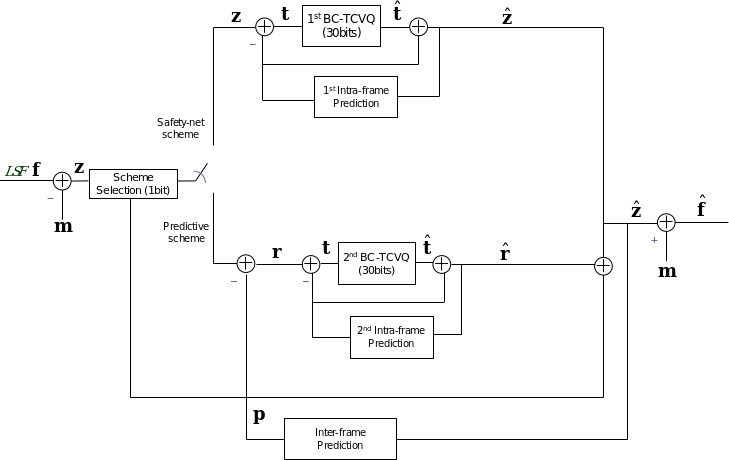Figure 23: Block diagram of the predictive BC-TCVQ with safety-net for an encoding rate of 31bits/frame

The operation of the 31 bit LSF quantizer is described as follows. If the safety-net scheme is selected, the mean-removed LSF vector, , is quantized by the 1st BC-TCVQ and 1st intra-frame prediction with 30 bits. If the predictive scheme is selected, the prediction error, , which is the difference between the mean-removed LSF vector and the prediction vector is quantized by the 2nd BC-TCVQ and 2nd intra-frame prediction with 30 bits.

An optimal index for each stage of BC-TCVQ is obtained by searching for an index which minimizes of equation (485).

, for =1,…, and =1,…, /2 (486)

where is the number of codevectors in the th sub-codebook, is the pth codevector of th the subcodebook, is a weighting function, and .

Intra-frame correlation typically remains in the inter-frame AR prediction error vectors. The presence of significant intra-frame correlation motivates the introduction of an intra-predictive coding scheme for the AR prediction error vector, as shown in figure 11, in order to increase the coding gain. The intra-frame prediction uses the quantized elements of the previous stage. The difference between and its prediction is then quantized. The prediction is formed for each trellis node using the output codevectors specified by the survivor path associated with the particular node.

The prediction coefficients used for the intra-frame prediction is predefined by the codebook training process. The prediction coefficients are two-by-two matrices for the 2-dimensional vector. The intra-frame prediction process of BC-TCVQ is as follows. The prediction residual vector, , which is the input of the 1st BC-TCVQ, is computed as

, for =1,…, /2-1 (487)

where

, for =1,…, /2-1 (488)

where is the estimation of , is the quantized vector of , and is the prediction matrix with 22 which is computed as

, for =1,…, /2-1, (489)

where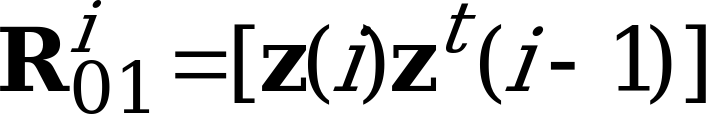and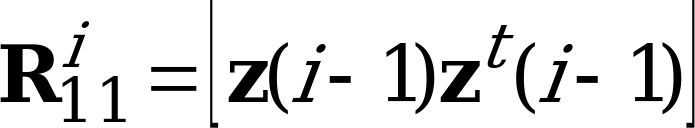(494)

and is the LPC order.

Then

, for =0,…, /2-1. (490)

The prediction residual, , is quantized by the 1st BC-TCVQ. The 1st BC-TCVQ and the 1st intra-frame prediction are repeated to quantize . Table 40 represents the designed prediction coefficients for the BC-TCVQ in the safety-net scheme.

Table 41: Intra-frame prediction coefficients for the BC-TCVQ in the safety-net scheme

 Coefficient Number Coefficient Value

For the predictive scheme, is quantized by the 2nd BC-TCVQ and the 2nd intra-frame prediction. An optimal index for each stage of BC-TCVQ is obtained by searching for an index which minimizes in equation (491).

The intra-frame prediction uses the same process with different prediction coefficients as that of the safety-net scheme. Then

, for i=0,…,M/2-1. (492)

The prediction residual, , is quantized by the 2nd BC-TCVQ. The 2nd BC-TCVQ and the 2nd intra-frame prediction are repeated to quantize. Table 42 represents the designed prediction coefficients for the BC-TCVQ in the predictive scheme.

Table 43: Intra-frame prediction coefficients for the BC-TCVQ in the predictive scheme

 Coefficient Number Coefficient Value

5.2.2.1.5.5 40 bit LSF quantization using the predictive BC-TCVQ/SVQ with safety-net

Figure 12 shows the predictive BC-TCVQ/split-VQ(SVQ) with safety-net for an encoding rate of 40 bits. Both 31 bit LSF quantizer and 40 bit LSF quantizer use the same codebook for BC-TCVQ.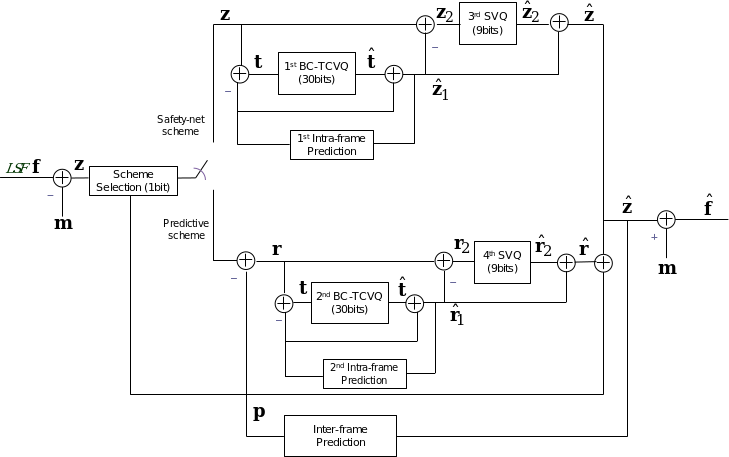Figure 13: Block diagram of the predictive BC-TCVQ/SVQ with safety-net for an encoding rate of 40 bits/frame

In the LSF quantization for an encoding rate of 40 bit/frame, the difference between the mean-removed LSF and its BC-TCVQ output is quantized by the 3rd and 4th SVQ, as shown in figure 14. The scheme selection, 1st and 2nd BC-TCVQ, and 1st and 2nd intra-frame prediction blocks of the 40 bit LSF quantizer are exactly same as those of the 31 bit LSF quantizer. Both LSF quantizers use same codebooks for the BC-TCVQ.

If the current coding mode in the scheme selection block is selected as the predictive scheme, the prediction error is derived by subtracting from the mean-removed LSF . It is quantized by the 2nd BC-TCVQ and the 2nd intra-frame prediction. The residual signal is obtained by subtracting from . The residual signal is then split into two sub-vectors of dimensions 8 and 8, and is quantized using the 4th SVQ. Since the low band is perceptually more important than the high band, five bits are allocated to the 1st 8-dimensional VQ and four bits are allocated to the 2nd 8-dimensional VQ. is quantized by the 4th SVQ to produce . is then obtained by adding to . Finally the predictive scheme output is derived by adding to .

If the current coding mode is selected as the safety-net scheme, the mean-removed LSF is quantized by the 1st BC-TCVQ and the 1st intra-frame prediction. The residual signal is extracted by subtracting from , and it is quantized by the 3rd SVQ to produce . The 3rd SVQ is exactly same as the 4th SVQ. That is, both SVQ quantizers use same codebooks. Because the input distribution of the 3rd SVQ is different from that of the 4th SVQ, scaling factors are used to compensate the difference. Scaling factors are computed by considering the distribution of both residual signals and . To minimize the computational complexity in in an actual implementation, the input signal of the 3rd SVQ is divided by the scaling factor, and the resulting signal is quantized by the 3rd SVQ. The quantized signal of the 3rd SVQ is obtained by multiplying the quantized output with the scaling factor. Table 44 shows the scaling factors for the quantization and de-quantization. Finally, the quantized mean-removed LSF is derived by adding to .

Table 45: Scaling factor for the SVQ

 Dimension 0 1 2 3 4 5 6 7 Inverse scale factor for quantization 0.5462 0.5434 0.5553 0.5742 0.58 0.5725 0.6209 0.6062 Scale factor for de-quantization 1.8307 1.8404 1.8009 1.7416 1.724 1.7467 1.6106 1.6497 Dimension 8 9 10 11 12 13 14 15 Inverse scale factor for quantization 0.6369 0.6432 0.6351 0.6173 0.6397 0.6562 0.6331 0.6404 Scale factor for de-quantization 1.5702 1.5548 1.5745 1.6199 1.5633 1.5239 1.5796 1.5615
5.2.2.1.6 Mid-frame LSF quantizer

For a more accurate representation of the spectral envelope during signal transitions, the encoder quantizes mid-frame LSF coefficients. In contrast to the frame-end LSF vector, the mid-frame LSF vector is not quantized directly. Instead, a weighting factor is searched in a codebook to calculate a weighted average between the quantized LSF vectors of the current and the previous frames. Only 2-6 bits are required depending on the bitrate and the coding mode (see Table 35a).

Table 35a: Bit allocation in mid-frame LSF quantization

 Bitrate [bps] IC UC VC GC TC AC 7200 2 5 4 5 5 2 8000 2 5 4 5 5 2 9600 2 5 4 5 0 0 13200 2 0 5 5 5 2 16400 4 0 5 5 0 0 24400 5 0 5 5 0 0 32000 5 0 0 5 5 5 64000 5 0 0 5 5 5

Before searching the codebook, the unquantized mid-frame LSF vector is weighted with the LSF weighting function defined in Equation (481). For simplicity, the following description will be provided by using LSP vectors instead of LSF vectors. These two vectors are related by the following simple relation where q(k) is the kth LSP coefficient and ω(k) is kth LSF coefficient. The mid-frame LSP weighting can be expressed using the following formula

, for k=0,…,M-1. (496a)

where is the kth unquantized LSP coefficient and is kth weighting factor of the function defined in Equation (481). Note, that this is not the weighting factor which is quantized. This weighting is based on the FFT spectrum where more weight is put on perceptually important part of the spectrum and less weight elsewhere.

The weighting factor to be quantized is a vector of size M that is searched in a closed-loop fashion such that the error between the quantized mid-frame LSP coefficients and this weighted representation is minimized in a mean-square sense. That is

(496b)

where is kth quantized weighted end-frame LSP coefficient and is the mid-frame weighting vector taken from the codebook. To save computation complexity, both operations are combined. That is

(496c)

Once the winning weighting factor is found, the quantized LSP vector is reordered to maintain a stable LP filter. After the quantization, the end-frame and the mid-frame LSF vectors are used to determine the quantized LP parameters in each subframe. This is done in the same way as for unquantized LP parameters (see Equation (58) in Clause 5.1.96).